# NEET 2020 Physics Paper With Solutions September 13

Get access to the complete set of questions from the Physics section of the NEET 2020 Question paper. 45 questions out of the total 180 questions are covered in Physics from syllabus of class 11 and 12 for NEET. Compared to the previous trends, Physics this year was easier as approximately 30-35% of the paper witnessed direct questions picked from NCERT sources. Calculations were not unusually lengthy. Mechanics was the unit which carried the highest weightage of all, 12 questions were asked from this unit alone.

### NEET 2020 - Physics (Sep 13)

Question 1. For transistor action, which of the following statements is correct?

1. a. Both emitter junctions as well as the collector junction are forward biased.
2. b. The base region must be very thin and lightly doped.
3. c. Base, emitter and collector regions should have same doping concentrations.
4. d. Base, emitter and collector regions should have same size.

Solution:

For a transistor action, the base junction must be lightly doped so that the base region is very thin.

Question 2. A spherical conductor of radius 10 cm has a charge of 3.2 × 10-7 C distributed uniformly. What is the magnitude of electric field at a point 15 cm from the centre of the sphere?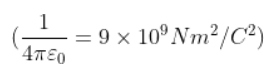1. a. 1.28 ×106 N/C
2. b. 1.28 ×107 N/C
3. c. 1.28 ×104 N/C
4. d. 1.28 ×105 N/C

Solution: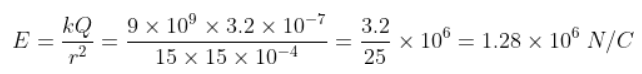Question 3. Assume that light of wavelength 600 nm is coming from a star. The limit of resolution of telescope whose objective has a diameter of 2m is:

1. a. 7.32 × 10–7 rad
2. b. 6.00 × 10–7 rad
3. c. 3.66 × 10–7 rad
4. d. 1.83 × 10–7 rad

Solution: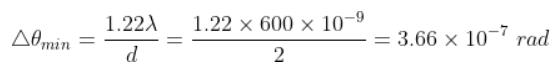Question 4. Dimensions of stress are:

1. a. [ML0T–2]
2. b. [ML–1T–2]
3. c. [MLT–2]
4. d. [ML2T–2]

Solution: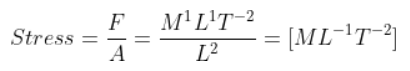Question 5. A screw gauge has least count of 0.01 mm and there are 50 divisions in its circular scale. The pitch of the screw gauge is:

1. a. 0.5 mm
2. b. 1.0 mm
3. c. 0.01 mm
4. d. 0.25 mm

Solution: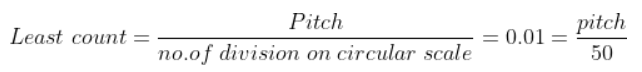Pitch = 0.01 × 50 = 0.5 mm

Question 6. Two bodies of mass 4 kg and 6 kg are tied to the ends of a massless string. The string passes over a pulley which is frictionless (see figure). The acceleration of the system in terms of acceleration due to gravity (g) is: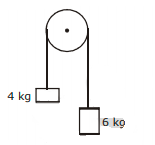1. a. g/5
2. b. g/10
3. c. g
4. d. g/2

Solution: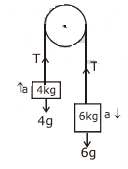6g – T = 6a

T – 4g = 4a

2g = 10a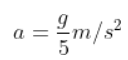Question 7. An electron is accelerated from rest through a potential difference of V volt. If the de Broglie wavelength of the electron is 1.227 × 10–2 nm, the potential difference is

1. a. 103 V
2. b. 104 V
3. c. 10 V
4. d. 102 V

Solution:

For e, according to de-Broglie's wavelength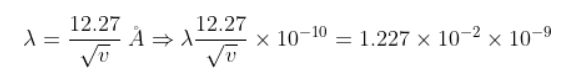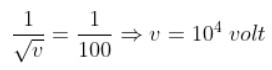Question 8. In a certain region of space with volume 0.2 m3, the electric potential is found to be 5 V throughout. The magnitude of electric field in this region is:

1. a. 1 N/C
2. b. 5 N/C
3. c. Zero
4. d. 0.5 N/C

Solution:

given v = const. (5 volt)

Therefore,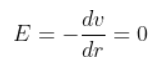Question 9. A cylinder contains hydrogen gas at pressure of 249 kPa and temperature 27°C.

Its density is: (R = 8.3 J mol–1 K–1)

1. a. 0.1 kg/m3
2. b. 0.02 kg/m3
3. c. 0.5 kg/m3
4. d. 0.2 kg/m3

Solution:

From ideal gas equation

∴ PV = nRT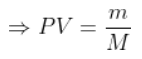∴ m = ρV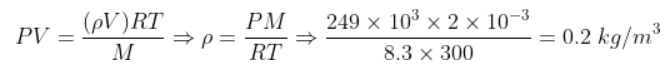Question 10. The mean free path for a gas, with molecular diameter d and number density n can be expressed as :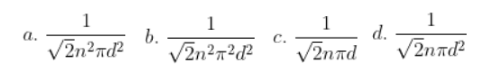Solution:

The mean free path for gas is given by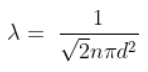Question 11. A ball is thrown vertically downward with a velocity of 20m/s from the top of a tower. It hits the ground after some time with a velocity of 80m/s. The height of the tower is :

(g= 10m/s2)

1. a. 320 m
2. b. 300 m
3. c. 360 m
4. d. 340 m

Solution: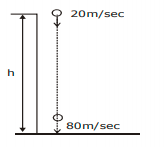v2 – u2 = 2as

(80)2  – (–20)2 = 2(–10) × s

6400 – 400 = 2 × (–10) × s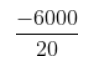= s

s = – 300 m

Height of tower h = 300 m

Question 12. For the logic circuit shown, the truth table is :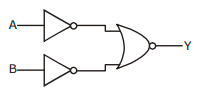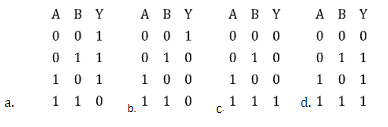Solution: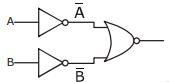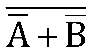=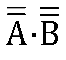= A.B (using de-Morgan’s rule)

∴ A B y = A.B

0 0 0

0 1 0

1 0 0

1 1 1

Question 13. A short electric dipole has a dipole moment of 16×10–9 C m. The electric potential due to the dipole at a point at a distance of 0.6 m from the centre of the dipole, situated on a line making an angle of 60° with the dipole axis is :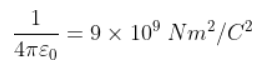1. a. 400 V
2. b. Zero
3. c. 50 V
4. d. 200 V

Solution: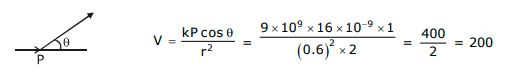Question 14. A capillary tube of radius r is immersed in water and water rises in it to a height h. The mass of the water in the capillary is 5g. Another capillary tube of radius 2r is immersed in water. The mass of water that will rise in this tube is :

1. a. 10.0 g
2. b. 20.0 g
3. c. 2.5 g
4. d. 5.0 g

Solution: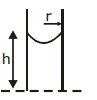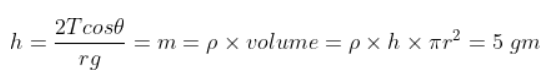r’ = 2r

∴h’ = h/2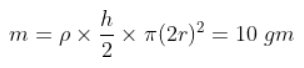Question 15. Which of the following graph represents the variation of resistivity (ρ) with temperature (T) For copper?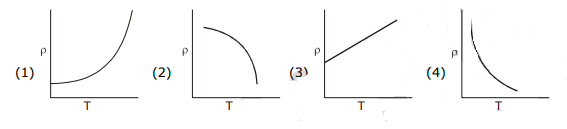Solution:

Relation between resistivity and temperature is given by ρ = ρ0e–αT

Question 16. The ratio of contributions made by the electric field and magnetic field components to the intensity of an electromagnetic wave is : (c = speed of electromagnetic waves)

1. a. 1 : c
2. b. 1 : c2
3. c. c : 1
4. d. 1 : 1

Solution:

Energy distribution is same so ration of electric field and magnetic field will be 1:1

Question 17. A long solenoid of 50 cm length having 100 turns carries a current of 2.5 A. The magnetic field at the centre of the solenoid is : (μ0 = 4π×10-7 Tm A-1)

1. a. 6.28×10-5T
2. b. 3.14×10-5T
3. c. 6.28×10-4T
4. d. 3.14×10-4T

Solution:

Magnetic field for solenoid is given by

B = μ0nI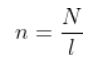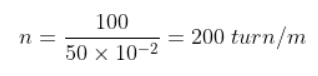= 4π × 10–7 × 200 × 2.5

= 4 × 3.14 × 10–7 × 500

= 2000 × 3.14 × 10–7

= 6.14 × 10–4 T

Question 18. For which one of the following, Bhor model is not valid?

1. a. Deuteron atom
2. b. Singly ionised neon atom (Ne+)
3. c. Hydrogen atom
4. d. Singly ionised helium atom (He+)

Solution:

Bohr Model is valid only for those atoms which have one electron in orbit.

Question 19. The energy equivalent of 0.5 g of a substance is :

1. a. 1.5×1013 J
2. b. 0.5×1013J
3. c. 4.5×1016J
4. d. 4.5×1013J

Solution:

E = Δmc2 =  0.5 × 10–3 × (3×108)2

= 0.5 ×10–3 × 9 ×1016

E = 4.5 ×1013 J

Question 20. Taking into account of the significant figures, what is the value of 9.99 m–0.0099 m?

1. a. 9.980 m
2. b. 9.9 m
3. c. 9.9801 m
4. d. 9.98 m

Solution:

9.99 - 0.0099 = 9.9801

As per rule

Least no. of place in decimal portion of any number

Ans. 9.98

Question 21. In a guitar, two strings A and B made of same material are slightly out of tune and produce beats of frequency 6 Hz. When tension in B is slightly decreased, the beat frequency increases to 7 Hz. If the frequency of A is 530 Hz, the original frequency of B will be :

1. a. 536 Hz
2. b. 537 Hz
3. c. 523 Hz
4. d. 524 Hz

Solution:

Frequency of A = fA = 530 Hz, fB=?

|fA – fB | = 6, given ⇒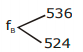When Tension B ↓ then fB↓, Beat Freq. ↑ to 7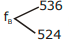But Beat Freq. ↑ 7

So if fB = 524 then Beat ↑ when fB↓

So fB = 524 Hz

Question 22. A series LCR circuit is connected to an AC voltage source. When L is removed from the circuit, the phase difference between current and voltage is π/3 . If instead C is removed from the circuit, the phase difference is again π/3 between current and voltage. The power factor of the circuit is :

1. a. 1.0
2. b. –1.0
3. c. zero
4. d. 0.5

Solution: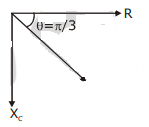When inductor alone is removed,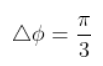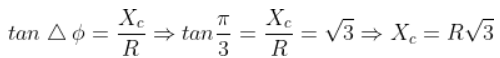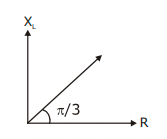When capacitor alone is removed,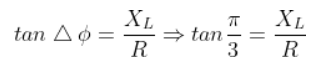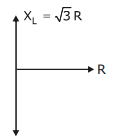Thus, for the original circuit,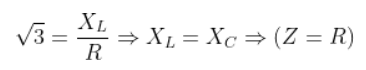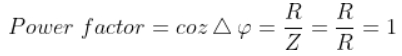Question 23. The quantities of heat required to raise the temperature of two solid copper spheres of radii r1 and r2 (r1 = 1.5 r2) through 1 K are in the ratio :

1. a. 3/2
2. b. 5/3
3. c. 27/8
4. d. 9/4

Solution:

Q = msΔT s is same as material is same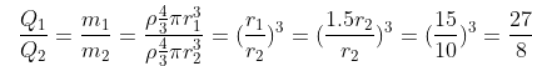Question 24. The Brewster’s angle ib for an interface should be :

1. a. 45° < ib < 90°
2. b. ib = 90°
3. c. 0° < ib < 30°
4. d. 30° < ib < 45°

Solution: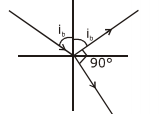m = tan (ib)

ib =  tan–1 (m)

(m> 1)

ib > 45

45 < ib < 90°

Question 25. Two cylinders A and B of equal capacity are connected to each other via a stop cock. A contains an ideal gas at standard temperature and pressure. B is completely evacuated. The entire system is thermally insulated. The stop cock is suddenly opened. The process is :

1. a. isochoric
2. b. isobaric
3. c. isothermal

Solution:

For the thermally insulated system,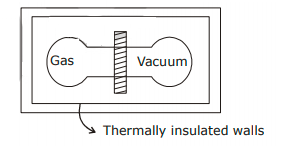⇒dQ = 0

and when the cock is removed

dw = 0

(Because in vacuum dw = 0)

⇒dQ = du + dw

So du also zero

Following are the essential conditions for the adiabatic process to take place. The system must be perfectly insulated from the surrounding. the process must be carried out quickly so that there is a sufficient amount of time for heat transfer to take place.

According to question both conditions satisfied so process will be adiabatic.

Question 26. An iron rod of susceptibility 599 is subjected to a magnetising field of 1200 A m-1. The permeability of the material of the rod is : (μ0 = 4π×10-7 T mA-1)

1. a. 2.4π ×10-5 T m A-1
2. b. 2.4π ×10-7 T m A-1
3. c. 2.4π ×10-4 T m A-1
4. d. 8.0×10-5 T m A-1

Solution:

xm = 599, μ0 = 4π × 10–7

H = 1200 A/m, μ= ?

μ = μ0 (1 + xm)

= 4π × 10–7 (1 + 599)

= 4π × 10–7 × 600

= 24π × 10–5

μ = 2.4π × 10–4TmA–1

Question 27. The capacitance of a parallel plate capacitor with air as medium is 6 μF. With the introduction of a dielectric medium, the capacitance becomes 30 μF. The permittivity of the medium is: (ε0 =8.85×10-12 C2 N-1 m-2)

1. a. 0.44×10-10 C2 N-1 m-2
2. b. 5.00 C2 N-1 m-2
3. c. 0.44×10-13 C2 N-1 m-2
4. d. 1.77×10-12 C2 N-1 m-2

Solution:

Cair = 6μF, Cmedium = 30μF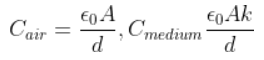Cmed = KCair

30μF = K6μF

K = 5

∴ε = ε0k = 8.85 × 10–12 × 5

ε = 44.25 × 10–12

ε = 0.4425 × 10–10

ε = 0.44 × 10–10 C2N–1m–2

Question 28. A charged particle having drift velocity of 7.5×10-4 m s-1in an electric field of3×10-10Vm–1 has a mobility in m2 V-1 s-1 of :

1. a. 2.5×10-6
2. b. 2.25×10-15
3. c. 2.25×1015
4. d. 2.5×106

Solution: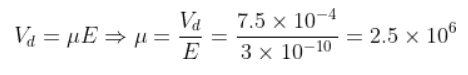(μ = mobility)

Question 29. The color code of a resistance is given below:The values of resistance and tolerance, respectively, are :

1. a. 4.7 kΩ, 5%
2. b. 470Ω, 5%
3. c. 470 kΩ, 5%
4. d. 47 kΩ, 10%

Solution: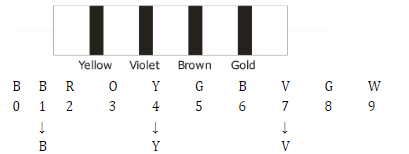R = 47 × 101Ω ± 5%

= 470 Ω ± 5%

So, 470, 5%

Question 30. The solids which have the negative temperature coefficient of resistance are :

1. a. semiconductors only
2. b. insulators and semiconductors
3. c. metals
4. d. insulators only

Solution:

Insulators have a negative temperature coefficient because as temperature increases, the resistance of insulators decreases. The resistivity of the semiconductor material to decrease with the rise in temperature, resulting in a negative temperature coefficient of resistance.

Question 31. A body weighs 72 N on the surface of the earth. What is the gravitational force on it, at a height equal to half the radius of the earth ?

1. a. 30 N
2. b. 24 N
3. c. 48 N
4. d. 32 N

Solution: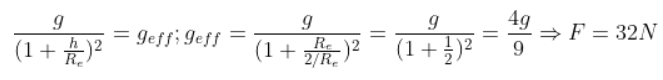Question 32. A 40 μF capacitor is connected to a 200 V, 50 Hz ac supply. The rms value of the current in the circuit is, nearly:

1. a. 2.5 A
2. b. 25.1 A
3. c. 1.7 A
4. d. 2.05 A

Solution:

Irms = Vrms/z = 200/Xc = 200. 2πf.C

= 200 × 2 × 3.14 × 50 × 40 × 10–6

Irms= 251.2 × 10–2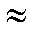2.5 A

Question 33. The phase difference between the displacement and acceleration of a particle in a simple harmonic motion is:

2. b. zero

Solution:

For SHM acceleration and displacement both must have opposite direction

a = –ω2x ∴ phase diff. is π

∴ x = A sin (ωt), a = –Aω2sinωt

Question 34. The average thermal energy for a mono-atomic gas is : (kB is Boltzmann constant and T, absolute temperature)

1. a. 5/2 kBT
2. b. 7/2 kBT
3. c. 1/2 kBT
4. d. 3/2 kBT

Solution:

By theory for monoatomic gas average thermal energy = 3/2 kBT

Question 35. Light of frequency 1.5 times the threshold frequency is incident on a photosensitive material. What will be the photoelectric current if the frequency is halved and intensity is doubled?

1. a. one-fourth
2. b. zero
3. c. doubled
4. d. four times

Solution:

f0< f1 = 1.5 f0

∴ f2 = 0.75 f0

for given condition

fincident<fthreshold

so no photo electron emission

i = 0

Question 36. A wire of length L, area of cross section A is hanging from a fixed support. The length of the wire changes to L1 when mass M is suspended from its free end. The expression for Young's modulus is :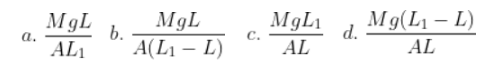Solution:

From young’s modulus formula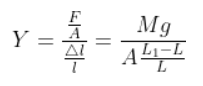Question 37. A ray is incident at an angle of incidence i on one surface of a small angle prism (with angle of prism A) and emerges normally from the opposite surface. If the refractive index of the material of the prism is μ, then the angle of incidence is nearly equal to: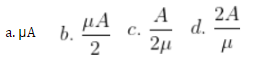Solution: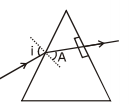1 sin i = μ. sin A

for small angle approximation

sinθ = θ

i = μA

Question 38. Find the torque about the origin when a force of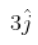N acts on a particle whose position vector is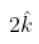m.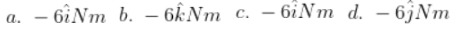Solution: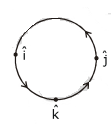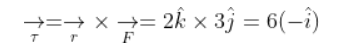Question 39. In Young's double slit experiment, if the separation between coherent sources is halved and the distance of the screen from the coherent sources is doubled, then the fringe width becomes :

1. a. four times
2. b. one-fourth
3. c. double
4. d. half

Solution:

Fringe width in YDSE is given by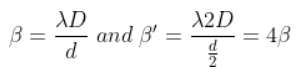Question 40. The energy required to break one bond in DNA is 10–20 J. This value in eV is nearly:

1. a. 0.06
2. b. 0.006
3. c. 6
4. d. 0.6

Solution:

1.6 × 10–19 Joule = 1 eV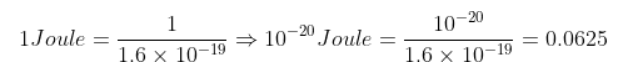Question 41. When a uranium isotope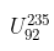is bombarded with a neutron, it generates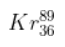, three neutrons and :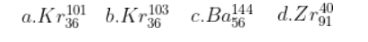Solution: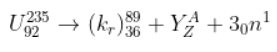92 = 36 + Z; A + 89 + 3 = 235 ⇒ A = 144

Z = 56

Question 42. Two particles of mass 5kg and 10 kg respectively are attached to the two ends of a rigid rod of length 1m with negligible mass. The centre of mass of the system from the 5kg particle is nearly at a distance of:

1. a. 67 cm
2. b. 80 cm
3. c. 33 cm
4. d. 50 cm

Solution: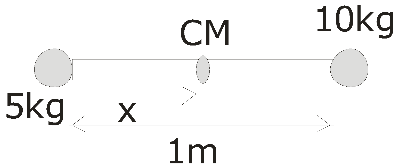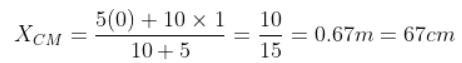Question 43. Light with an average flux of 20 W/cm2 falls on a non-reflecting surface at normal incidence having surface area 20 cm2. The energy received by the surface during time span of 1 minute is:

1. a. 24 × 103 J
2. b. 48 × 103 J
3. c. 10 × 103 J
4. d. 12 × 103 J

Solution:

energy/sec = 20 w/cm2 × 20 cm2

= 400 w

∴ in 1 minute = 400 × 60

= 24000

Question 44. The increase in the width of the depletion region in a p-n junction diode is due to:

1. a. both forward bias and reverse bias
2. b. increase in forward current
3. c. forward bias only
4. d. reverse bias only

Solution:

The increase in the width of the depletion region in a p-n junction diode is due to reverse bias only.

Question 45. A resistance wire connected in the left gap of a metre bridge balances a 10 Ω resistance in the right gap at a point which divides the bridge wire in the ratio 3:2. If the length of the resistance wire is 1.5 m, then the length of 1Ω of the resistance wire is:

1. a. 1.5 × 10–1 m
2. b. 1.5 × 10–2 m
3. c. 1.0 × 10–2 m
4. d. 1.0 × 10–1 m

Solution: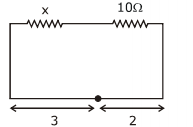x × 2λ = 10 × 3 λ

x = 15Ω

∴ 15Ω→ 1.5 m

∴ 1Ω→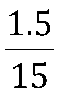= 1 × 10–1 m

### NEET 2020 Physics Paper With Solutions September 13